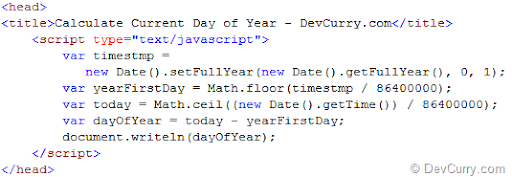# JavaScript: Find Day of Year

 Tweet
Here’s a simple script that finds the current day of the yearWe first get the timestamp for the 1st day of the year - number of seconds elapsed since midnight Coordinated Universal Time (UTC) of January 1, 1970, not counting leap seconds. This is done using the following piece of code

var timestmp = new Date().setFullYear(new Date().getFullYear(), 0, 1);

The setFullYear() takes the year, month and day as a parameter. Since month in JavaScript ranges from 0 – 11, we have given the month as 0 i.e. January. The day is set to 1. For the year, we are doing new Date().getFullYear() which returns the current year 2011.

We then divide this timestamp by the total number of seconds in a day – 86400000. This gives us the number of days on Jan 1st of the current year, since the Unix epoch at January 1st 1970 midnight UTC

var yearFirstDay = Math.floor(timestmp / 86400000);

The next line calculates number of days since the Unix epoch at January 1st, 1970 midnight UTC till the current date, that is today.

var today = Math.ceil((new Date().getTime()) / 86400000);

At the end, we calculate the current day of the year by subtracting first day of the year – today.

var dayOfYear = today - yearFirstDay;

OUTPUT of the code if run today, is 217.